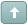Error

 Previous Topic Next Topic
MS Support
#1 Posted : Tuesday, November 5, 2019 6:20:13 PM(UTC)

Groups: Moderators, Registered, Registered Users, Subscribers
Joined: 10/8/2010(UTC)
Posts: 1,631

Thanks: 52 times
Was thanked: 130 time(s) in 126 post(s)

Anthony Garner's article, “Backtesting a Mean-Reversion Strategy in Python” presented a trading system and the code to backtest it using the Python language. The code to test the system in MetaStock is provided here.

# General tab:

Name: Mean-Reversion Strategy

Notes:

Based on code provided by Anthony Garner

Formula:

Code:
``````length:= 10;
sma:= mov(c,length, S);
lma:= mov(c, length*10, s);
dev:= std(c, length);
z:= (C - sma)/dev;
(z < -1) AND (sma > lma)``````

# Sell Order tab:

Formula:

Code:
``````length:= 10;
sma:= mov(c,length, S);
lma:= mov(c, length*10, s);
dev:= std(c, length);
z:= (C - sma)/dev;
z > -0.5``````

# Sell Short Order tab:

Formula:

Code:
``````length:= 10;
sma:= mov(c,length, S);
lma:= mov(c, length*10, s);
dev:= std(c, length);
z:= (C - sma)/dev;
(z > 1) AND (sma < lma)``````

# Buy To Cover Order tab:

Formula:

Code:
``````length:= 10;
sma:= mov(c,length, S);
lma:= mov(c, length*10, s);
dev:= std(c, length);
z:= (C - sma)/dev;
z < 0.5``````

 Wanna join the discussion?! Login to your Discussions forum accountor Register a new forum account.Users browsing this topic
Forum Jump
You cannot post new topics in this forum.
You cannot reply to topics in this forum.
You cannot delete your posts in this forum.
You cannot edit your posts in this forum.
You cannot create polls in this forum.
You cannot vote in polls in this forum.